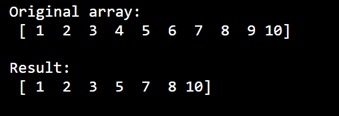# How to remove specific elements from a NumPy array?

Learn, how to remove specific elements from a NumPy array?
Submitted by Pranit Sharma, on December 30, 2022

NumPy is an abbreviated form of Numerical Python. It is used for different types of scientific operations in python. Numpy is a vast library in python which is used for almost every kind of scientific or mathematical operation. It is itself an array which is a collection of various methods and functions for processing the arrays.

## Removing specific elements from a NumPy array

Suppose we are given a NumPy array with 10 elements and we need to delete some specific elements from this array (say 3,5,8).

For this purpose, we will use numpy.delete() method. This method returns a new array with sub-arrays along an axis deleted.

To delete some specific elements from an array, we will pass the list of indices of those elements which we want to remove.

Let us understand with the help of an example,

## Python code to remove specific elements from a NumPy array

```# Import numpy
import numpy as np

# Import pandas
import pandas as pd

# Creating a numpy array
arr = np.array([1, 2, 3, 4, 5, 6, 7, 8, 9,10])

# Display original array
print("Original array:\n",arr,"\n")

# Defining a list of indices
ind = [3,5,8]

# Deleting specific elements
res = np.delete(arr,ind)

# Display result
print("Result:\n",res,"\n")
```

Output: